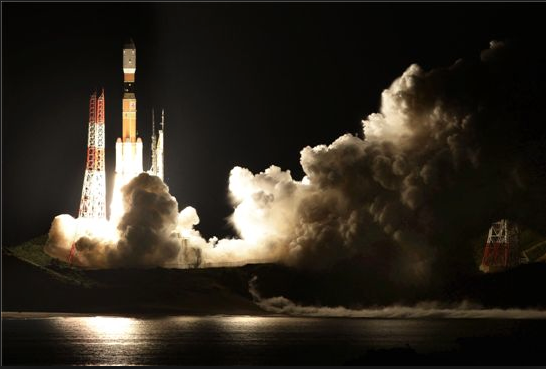# Net force to a rocketA $500 \text{-kg}$ rocket is to be accelerated from rest to $1600 \text{ km/h}$ at a constant rate in $1.8$ seconds. What is the approximate magnitude of the required net force?

Assume the loss of fuel makes a negligible change to the mass of the rocket.

×